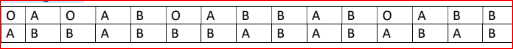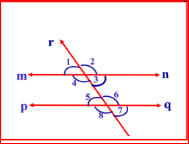# Karnataka Class 9 Maths Important Questions

Karnataka Class 9 Maths, mostly follow closely with the Class 10 curriculum. Hence, a student who does well in Class 9 Maths, will find it easy with the higher classes. The syllabus prescribed by the Karnataka Secondary Education Examination Board is strictly followed in each of the classes. According to the prescribed syllabus, the Maths chapters are well organised and covers important topics such as Number System, Geometry, Lines and Angles, Polynomials, Triangles and Probability and more. Now, the subject is interesting and students who wish to score high will definitely need a lot of practice and they get it with the help of not just Class 9 Karnataka State Board Question Papers but also the Karnataka Class 9 Math Important Questions.

Here, there is a list of important questions collected by BYJU’S so that we can provide students with support.

Class 9 Maths Important Questions

1) Prove that in circle, equal chord forms equal angles at the centre

2) Construct a triangle ABC if BC = 7cm, ∠B = 75 and AB+AC = 13cm

3) solve graphically x + y = 7

4) Give one example each for quadratic and linear polynomials .

5) Draw a circle of radius 2.5cm mark Diameter and chord.

6) Calculate area of triangle whose sides are of the length 5 cm , 4 cm, 3cm

7) Blood group of 30 students is given below Construct frequency distribution table for following data:8) Expand By using suitable identity : (x+4) (X+10)

9) Prove that in a circle line Drawn thrown the centre of the circle to bisect a chord is

perpendicular to the chord.

10) Prove that angles opposite to equal sides of an isosceles triangle are equal.

11) The cost of a book is twice the cost of a pen. Write a linear equation in two variables to represent.

1. x-2y=0 (b) x+2y=0 © 2x-2y=0 (d) 3x-2y=0

12) State SAS Congruence Rule:

14) Find the decimal expansion of

$$\begin{array}{l}\frac{10}{3}\end{array}$$

15) Write any four Euclid’s axioms

16) In the below figure, write one pair of:1. Corresponding angles
2. Alternate interior angles

17) In a cricket match, a batswoman hits a boundary 6 times out of 30balls she plays. Find the probability that She did not hit a boundary.

18) A cylindrical pillar is 50cm in diameter and 3.5m in height. Find the cost of painting the curved surface of the pillar at the rate of Rs. 12.50 per

$$\begin{array}{l}m^{2}\end{array}$$
.

19) Find the volume of your Geometric Box

20) The taxi fare in a city is as follows: for the first kilometre, the fare is Rs.8 and for the subsequent distance. It is Rs. 5 per km. Taking the distance covered as x km and total fare as Rs y, write a linear equation for this Information, and draw its graph.

### Why to Solve Karnataka Board Class 9 Maths Important Questions?

Well, so that you know where to find them, why not start solving them? It will definitely help you all. See, here some advantages of doing these important questions.

• Questions cover important topics of the subject as per syllabus
• It gives an idea of the repeating questions and trends
• Students can prepare well for the exams
• Students gain practice and is confident to face exams

For more such help and resources of karnataka sslc examination board also, students can reach out to BYJU’S.